Courses

# Influence Line Diagram and Rolling Loads Civil Engineering (CE) Notes | EduRev

## GATE : Influence Line Diagram and Rolling Loads Civil Engineering (CE) Notes | EduRev

The document Influence Line Diagram and Rolling Loads Civil Engineering (CE) Notes | EduRev is a part of the GATE Course Structural Analysis II.
All you need of GATE at this link: GATE

Q.1 A long uniformly distributed load of 10 kN/m and a concentrated load of 60 kN are moving together on the beam ABCD shows in the figure (not drawn to scale). The relative positions of the two loads are not fixed. The maximum shear force (in kN, round off to the nearest integer) caused at the internal hinge B due to the two loads is______    [2019 : 2 Marks, Set-I I]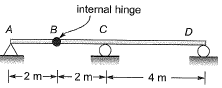Solution:

ILD for VB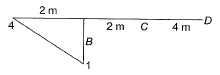Max. shear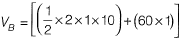= 70KN
Note: The maximum shear force at hinge B may be +70 kN or -70 kN. So, student can challenge this questions.

Q.2 Consider the beam ABCD shown in the figure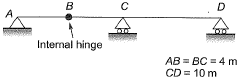For a moving concentrated load of 50 kN on the beam, the magnitude of the maximum bending moment (in kN-m) obtained at the support C will be equal to _________ .    [2017 : 2 Marks, Set-I]
Solution: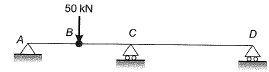ILD for BM at C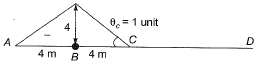Max. BM occurs at C, when 50 kN load is at B.
Max. BM at C = 50 x (-4) = -200 kNm

Q.3 A simply supported beam AB of span, L = 24 m is subjected to two wheel loads acting at a distance, d = 5 m apart as shown in the figure below. Each wheel transmits a load, P = 3 kN and may occupy any position along the beam. If the beam is an I-section having section modulus, S=16.2 cm3, the maximum bending stress (in GPa) due to the wheel loads is _________ .    [2015 : 2 Marks, Set-II]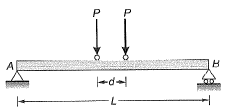Solution:

Method-1 (By ILD)
C.G. of system = 2.5 m from any load
For maximum bending moment, system of load should be,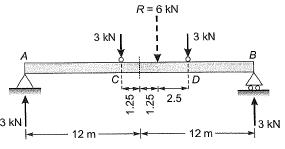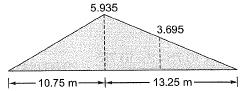Maximum BM will occur below load at C. Ordinate of ILD at C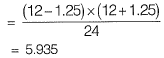Ordinate of ILD at D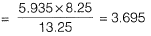Maximum BM = 5.935 x 3 + 3.695 x 3
= 28.89 kN-m
Maximum bending stress,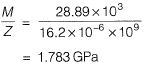Method-II  (By Statics)
Let the section at which absolute max. BM ocurs be at x distance from left support.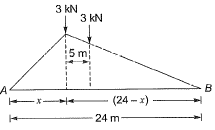Max. BM at section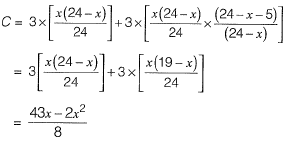For absolute max. BM,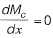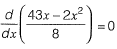⇒ x = 10.75 m
Absolute max BM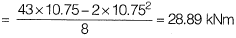Absolute max bending stress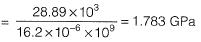AVOID MISTAK
(I)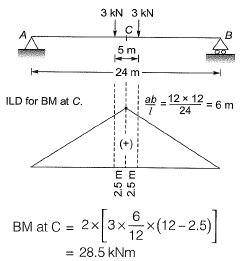Max. bending stress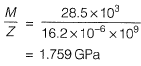(II)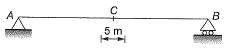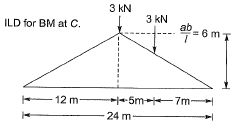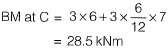Max. bending stress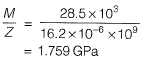This is the maximum bending stress at mid= span and not the absolute max. bending stress in the beam.

Q.4 In a beam of length L, four possible influence line diagrams for shear force at a section located at a distance of L/4  from the left end support (marked as P, Q, R and S) are shown below. The correct influence line diagram is    [2014 : 1 Mark, Set-I]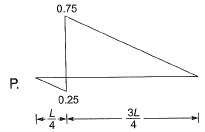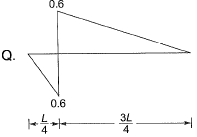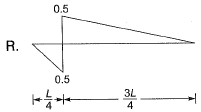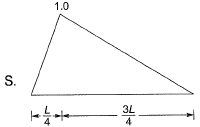(a) P
(b) Q
(c) R
(d) S
Ans.
(A)
Solution: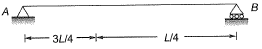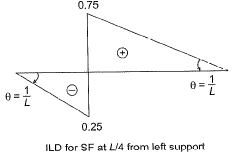Q.5 Beam PQRS has internal hinges in spans in spans PQ and RS as shown. The beam may be subjected to a moving distributed vertical load of maximum intensity 4 kN/m of any length anywhere on the beam. The maximum absolute value of the shear force (in kN) that can occur due to this loading just to the right of support Q shall be   [2013 : 2 Marks]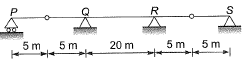(a) 30 kN
(b) 40 kN
(c) 45 kN
(d) 55 kN
Ans.
(C)
Solution:
Drawing the ILD of shear force just to right of Q by using Muller Breslau’s principle. A cut is made just to the right of Q, since cut is very close to support Q, therefore displacement of left portion is almost zero and that to the right portion will be 1.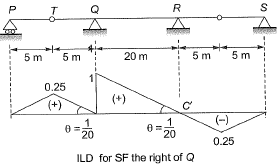If moving distributed load is present over span PR then we get maximum shear force just to the right of Q.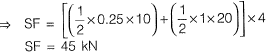Offer running on EduRev: Apply code STAYHOME200 to get INR 200 off on our premium plan EduRev Infinity!

## Structural Analysis II

109 docs|22 tests

,

,

,

,

,

,

,

,

,

,

,

,

,

,

,

,

,

,

,

,

,

;